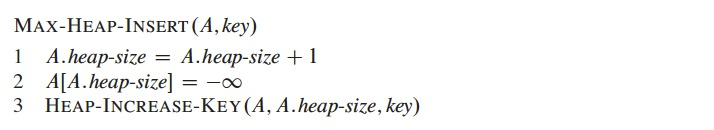July 17, 2019

# Heapsort

• Running time $$O(n \lg n)$$, like merge sort
• Sorts in place (as insertion sort), only constant number of array elements are stored outside the input array at any time
• Combines better attributes of these other sorting algorithms
• Uses an algorithm design technique: using a data structure, a heap
• Heap also referred as garbage-collected storage
• As in java and Lisp

# Heapsort

• Binary heap data structure
• Array object can be viewed as a nearly complete binary tree
• Each node corresponds to an element of the array
• Tree completely filled on all levels, except (possibly) the lowest
• Lowest filled from the left up to a point

# Heapsort

• Binary heap data structure
• An array $$A$$ represents the heap, an object with two attributes
• $$A.length$$, number of elements in the array
• $$A.heap-size$$, how many elements in the heap are stored within array $$A$$
• $$A[1 .. A.length]$$ may contain numbers but only elements in $$A[1 .. A.heap-size]$$, where $$0 \leq A.heap-size \leq A.lenght$$, are valid elements in the heap
• Root of tree is $$A$$
• Given index $$i$$ of a node, we can compute indices of its parent, left child, and right child

# Heapsort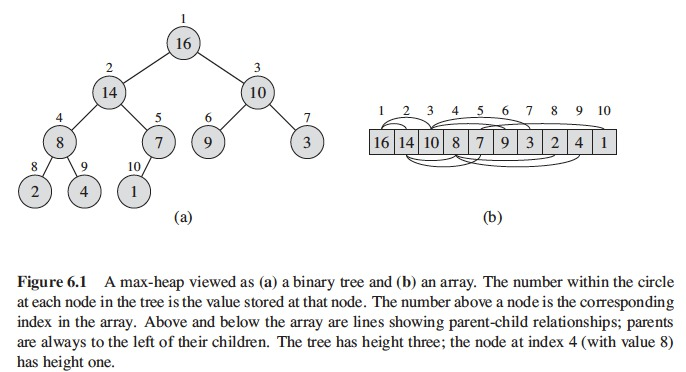# Heapsort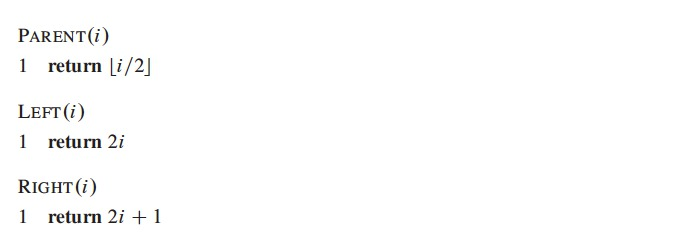# Heapsort

• Two kinds of binary heaps
• The values in the nodes satisfy the heap property
• max-heaps
• max-heap property
• For every node $$i$$ other than the root, $$A[PARENT(i)] \geq A[i]$$
• The value of a node is at most the value of its parent
• Largest element in a max-heap is stored at the root

# Heapsort

• Two kinds of binary heaps
• min-heaps
• min-heap property
• For every node $$i$$ other than the root, $$A[PARENT(i)] \leq A[i]$$
• The smallest element in a min-heap is at the root

# Heapsort

• max-heaps are used for the heapsort algorithm
• min-heaps implement priority queues
• Viewing heap as a tree
• height of a node in a heap $$\rightarrow$$ number of edges on the longest simple downward path from the node to a leaf
• height of a heap $$\rightarrow$$ the height of its root
• A heap of $$n$$ elements based on a complete binary tree
• Height is $$\Theta( \lg n)$$
• Basic operations on heaps run in time proportional to the height of the tree
• $$O( \lg n)$$

# Heapsort

• MAX-HEAPIFY, runs in $$O( \lg n)$$ time, key to mantain the max-heap property
• BUILD-MAX-HEAP, linear time, produces a max-heap from an unordered input array
• HEAPSORT, runs in $$O(n \lg n)$$ time, sorts an array
• MAX-HEAP-INSERT, HEAP-EXTRACT-MAX, HEAP-INCREASE-KEY, and HEAP-MAXIMUM, run in $$O( \lg n)$$ time
• Used to implement a priority queue

# Maintaining the Heap Property

• MAX-HEAPIFY used to maintain the max-heap property
• Inputs: an array $$A$$ and an index $$i$$
• MAX-HEAPIFY assumes that binary trees rooted at LEFT(i) and RIGHT(i) are max-heaps, but that $$A[i]$$ might be smaller than its children (violating the max-heap property)
• MAX-HEAPIFY moves the value $$A[i]$$ down in the max-heap so that subtree rooted at index $$i$$ follows the max-heap property

# Maintaining the Heap Property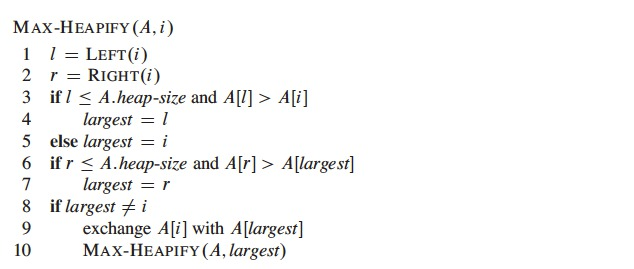# Maintaining the Heap Property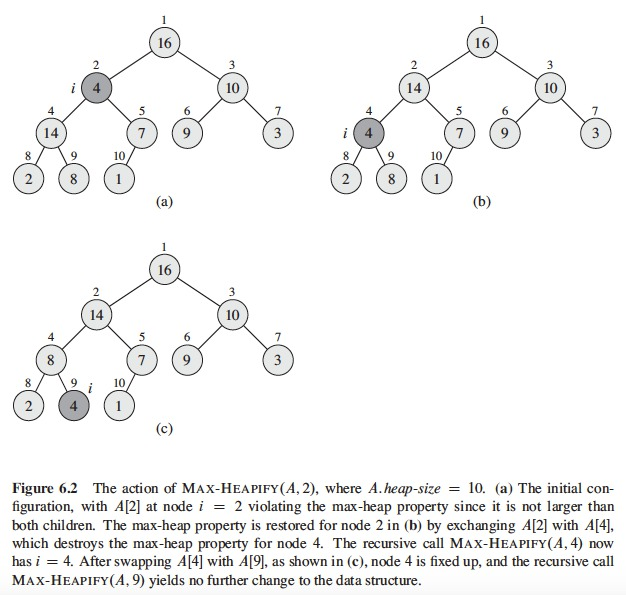# Maintaining the Heap Property

• Running time of MAX-HEAPIFY on subtree of size $$n$$ at node $$i$$ is
• $$\Theta(1)$$ to fix up relationships among $$A[i]$$, $$A[LEFT(i)]$$, and $$A[RIGHT(i)]$$
• Time to run MAX-HEAPIFY on subtree rooted at one children of node $$i$$
• Children’s subtrees have size at most $$2n/3$$
• Worst case when bottom level of tree is exactly half full
• Running time of MAX-HEAPIFY with recurrence
• $$T(n) \leq T(2n/3) + \Theta(1)$$
• Solution to recurrence by case 2 of master theorem
• $$T(n) = O( \lg n)$$

# Building a Heap

• Use MAX-HEAPIFY bottom-up
• Convert array $$A[1 .. n]$$, $$n = A.length$$, into a max-heap
• Elements in the subarray $$A[(\lfloor n/2 \rfloor + 1) .. n]$$ are the leaves of the tree
• Each of these is a 1-element heap to begin with
• BUILD-MAX-HEAP($$A$$) goes through remaining nodes of the tree
• Runs MAX-HEAPIFY on each of them

# Building a Heap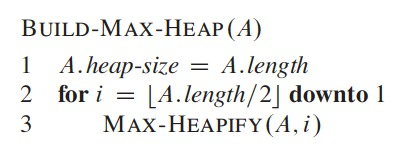# Building a Heap# Building a Heap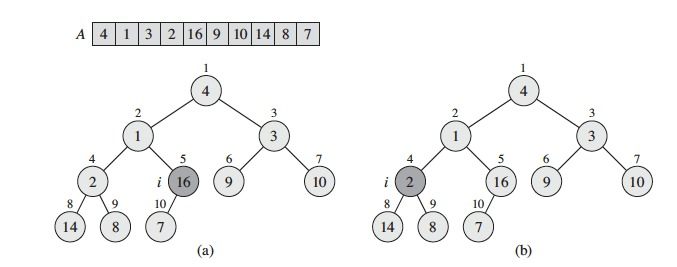# Building a Heap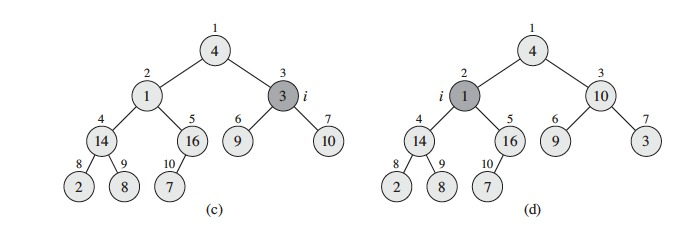# Building a Heap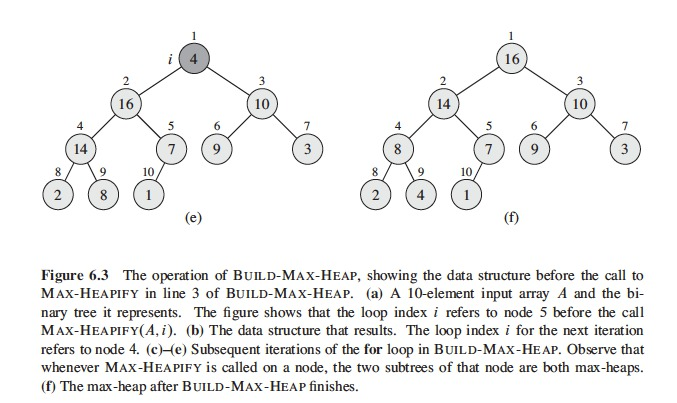# Loop Invariant for BUILD-MAX-HEAP

• Start of each iteration of for loop, lines 2-3, each node $$i + 1$$, $$i + 2$$, $$\dots$$, $$n$$ is the root of a max-heap
• Loop invariant shows
• This invariant is true prior to first loop iteration
• Each iteration of loop maintains the invariant
• The invariant provides useful property to show correctness when the loop terminates

# Loop Invariant for BUILD-MAX-HEAP

• Initialization: Prior to first iteration of loop
• $$i = \lfloor n/2 \rfloor$$.
• Each node $$\lfloor n/2 \rfloor + 1$$, $$\lfloor n/2 \rfloor + 2$$, $$\dots$$, $$n$$ is a leaf and also the root of a trivial max-heap

# Loop Invariant for BUILD-MAX-HEAP

• Maintenance: See that each iteration maintains the loop invariant
• Children of node $$i$$ numbered higher than $$i$$, they are both roots of max-heaps
• This is required by MAX-HEAPIFY($$A$$, $$i$$) to make node $$i$$ a max-heap root
• MAX-HEAPIFY($$A$$, $$i$$) preserves property that nodes $$i + 1$$, $$i + 2$$, $$\dots$$, $$n$$ are all roots of a max-heap
• Decreasing $$i$$ in for loop update, reestablishes loop invariant for next iteration

# Loop Invariant for BUILD-MAX-HEAP

• Termination: at termination $$i = 0$$
• By loop invariant, each node 1, 2, $$\dots$$, $$n$$ is the root of a max-heap
• In particular, node 1, the root of the tree

# Upper Bound for BUILD-MAX-HEAP

• Each call to MAX-HEAPIFY costs $$O( \lg n)$$ time
• BUILD-MAX-HEAP makes $$O(n)$$ such calls
• Running time is $$O(n \lg n)$$
• This upper bound is correct but not asymptotically tight

# A Tighter Bound for BUILD-MAX-HEAP

• Time for MAX-HEAPIFY to run at a node varies with height of the node in the tree
• Heights of most nodes are small
• Tighter analysis uses properties that an $$n$$-element heap has height $$\lfloor lg n \rfloor$$ and at most $$\lceil n/2^{h+1} \rceil$$ nodes of any height $$h$$
• Time required by MAX-HEAPIFY when called on node of height $$h$$ is $$O(h)$$
• Total cost of BUILD-MAX-HEAP: $$\sum_{h=0}^{\lfloor \lg n \rfloor} \lceil \frac{n}{2^{h+1}} \rceil O(h)$$ $$= O \left( n \sum_{h=0}^{\lfloor \lg n \rfloor} \frac{h}{2^h} \right)$$.

# A Tighter Bound for BUILD-MAX-HEAP

• Evaluating summation by substituting $$x = 1/2$$ in formula (A.8)

$$\sum_{h=0}^{\infty} \frac{h}{2^h} = \frac{1/2}{(1 - 1/2)^2} = 2$$

• We bound running time of BUILD-MAX-HEAP as

$$O \left( n \sum_{h=0}^{\lfloor \lg n \rfloor} \frac{h}{2^h} \right)$$ $$=O \left( n \sum_{h=0}^{\infty} \frac{h}{2^h} \right)$$ $$=O(n)$$

# Heapsort

• Starts by using BUILD-MAX-HEAP on input array $$A[1 .. n]$$, where $$n = A.length$$
• Maximum element of array stored at root $$A$$, we can put it in its final position, exchanging it by $$A[n]$$
• Now, the new root might violate the max-heap property, we restore it by calling MAX-HEAPIFY($$A$$, 1), that leaves a max-heap $$A[1 .. n-1]$$
• The process is repeated for max-heap size $$n-1$$ down to a heap of size 2

# Heapsort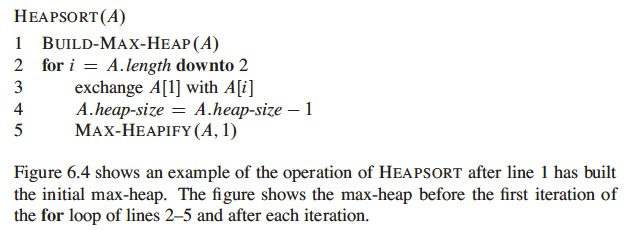# Heapsort

• Running time: $$O(n \lg n)$$
• Call to BUILD-MAX-HEAP takes time $$O(n)$$
• Each of $$n-1$$ calls to MAX-HEAPIFY take $$O( \lg n)$$

# Heapsort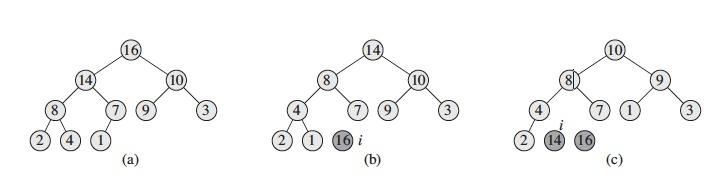# Heapsort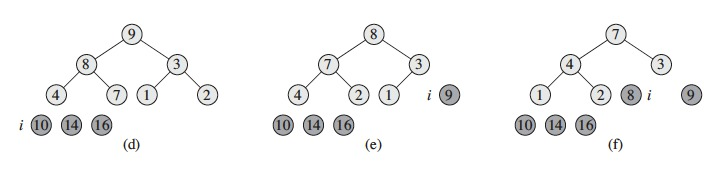# Heapsort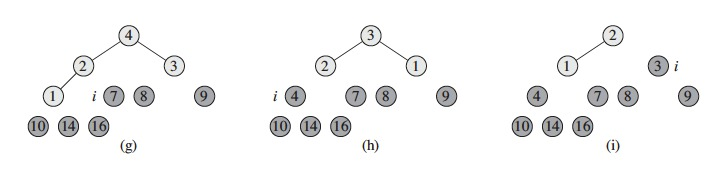# Heapsort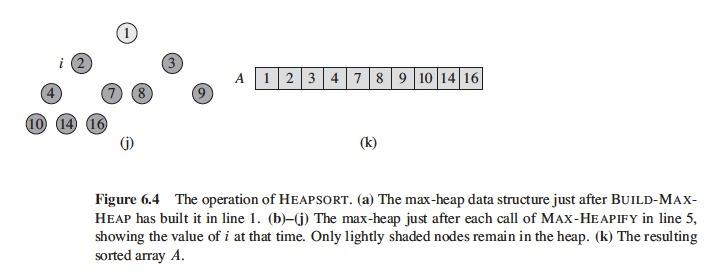# Priority Queues

• Heapsort is a good sorting algorithm but quicksort usually beats it in practice
• The heap data structure has many uses
• A popular application of heaps is an efficient priority queue
• A priority queue can be max-priority or min-priority
• We focus on max-priority queues, based on max-heaps

# Priority Queues

• A priority queue is a data structure that mantains a set $$S$$ of elements, each with an associated value called a key. A max-priority queue supports the operations
• INSERT($$S, x$$): inserts element $$x$$ into set $$S$$, equivalent to $$S = S \cup \{x\}$$
• MAXIMUM($$S$$): returns the element $$S$$ with the largest key
• EXTRACT-MAX($$S$$): removes and returns the element of $$S$$ with the largest key
• INCREASE-KEY($$S, x, k$$): increases the value of element $$x$$’s key to new value $$k$$, which is assumed to be as large as $$x$$’s current value

# Priority Queues

• HEAP-MAXIMUM runs in $$\Theta(1)$$ time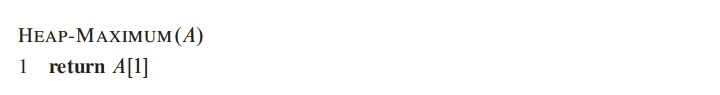# Priority Queues

• HEAP-EXTRACT-MAX runs in $$O( \lg n)$$ time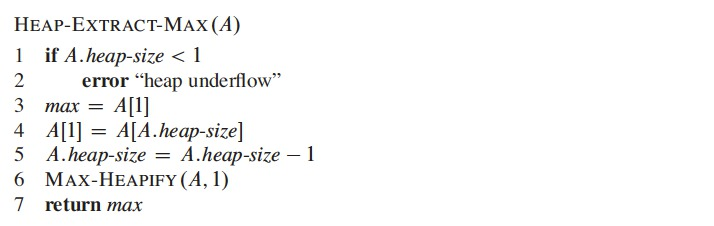# Priority Queues

• HEAP-INCREASE-KEY runs in $$O( \lg n)$$ time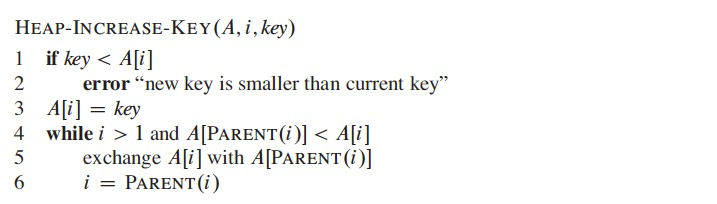# Priority Queues

• MAX-HEAP-INSERT runs in $$O( \lg n)$$ time